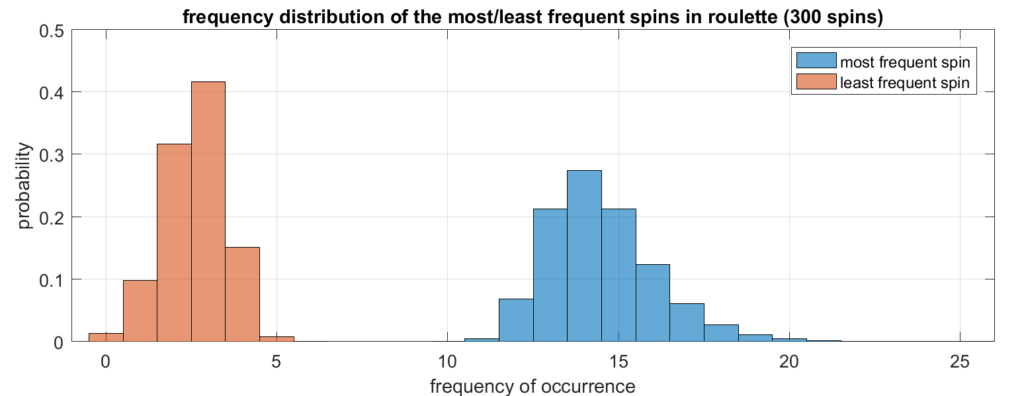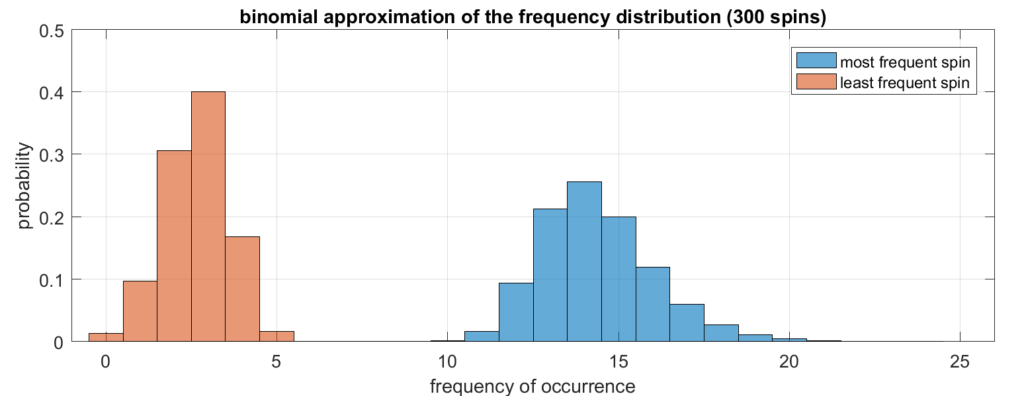## Lucky spins in rouletteRoulette is a game where the dealer spins a numbered wheel containing a marble. Once the wheel settles, the marble lands on exactly one (winning) number. A standard American roulette wheel has 38 numbers on it. Players may place bets on a particular number, a color (red or black), or a particular quadrant of the wheel.I was recently in Las Vegas for a conference, and I noticed that the roulette tables in the conference hotel were equipped with screens that display real-time statistics! A computer keeps track of the winning numbers for the past 300 spins and shows how frequently each number was a winner (see photo on the right). The two “elite numbers” were 12 and 27 (each occurred 13 times in the past 300 games) and the “poor performer” was 0, which only occurred 2 times.

Even if the roulette wheel is equally likely to land on each number, there will be natural fluctuations. Some numbers will obviously win more frequently than others in any finite number of spins. But what sorts of deviations can we expect? In the picture above, the spread between most frequent winner and least frequent winner was 13 to 2 over the course of 300 games. Is this normal? In other words, how big would the spread have to be before you suspected that the roulette wheel was rigged and was favoring some numbers over others? Let’s find out!

### Results via simulation

Let’s suppose the wheel has $n$ different numbers on it, which we label $\{1,2,\dots,n\}$, and we spin it $m$ times when we aggregate our statistics. Note that in the actual roulette game, $n=38$ and $m=300$. If the game is fair, then each number is equally likely to occur and the spins are mutually independent. In other words, rolling a 4 on your first spin doesn’t make you more or less likely to roll a 4 or any other number on subsequent spins.

This sort of game is straightforward to simulate in Matlab. I wrote code that plays 300 games, counts how many times the most and least frequent numbers occur, and then repeats the entire process a large number of times. Then, I plotted a histogram showing what proportion of the time the most frequent number occurred 10 times, 11 times, etc. This is known as the empirical distribution. Here are the results of the simulation (aggregated over 100 million trials).As we can see, a spread from 2 to 13 is right around what we expect! Of course, outliers are still possible. For example, it’s possible for the luckiest number to win 30 times out of 300 spins, but this only happened twice out of 100,000,000 simulated runs.

### Analytical results

The roulette wheel lands on one of the $n$ numbers with equal probability. We then perform $m$ spins and count how many times each number occurs. If we place the counts in the vector $(x_1,\dots,x_n)$, where $x_1+\dots+x_n=m$, then this vector follows a multinomial distribution. The probability mass function is therefore:
$p(x_1,\dots,x_n) = {m \choose {x_1,\dots,x_m}} \frac{1}{n^m}$The bracketed term is called a multinomial coefficient and it counts the number of ways we can spin a “$1$” $x_1$ times, a “$2$” $x_2$ times, and so on. This also corresponds to the number of ways of depositing $m$ distinct objects into $n$ distinct bins, with $x_1$ objects in the first bin, $x_2$ objects in the second bin, and so on. It’s also equal to $\frac{m!}{x_1! \cdot x_2!\cdots x_n!}$. Meanwhile, $n^m$ is the number of different ways we can spin the roulette wheel $m$ times.

The probability that the most frequent spin occurs at most $k$ times is:
$F_\text{max}(k) = \sum_{\substack{ x_1+\dots+x_n=m\\x_i\,\le\,k}} p(x_1,\dots,x_n)$In other words, we must sum over all tuples $(x_1,\dots,x_n)$ of nonnegative integers that sum to $m$, that are each no greater than $k$. This is the cumulative distribution function, and in order to obtain the probability that the most frequent spin occurs exactly $k$ times (the probability mass function), we compute the difference:
$q_\text{max}(k) = F_\text{max}(k)-F_\text{max}(k-1)$A similar expression can be obtained for the probability that the least frequent spin occurs exactly $k$ times. These sums are impractical to compute because they have an astronomical number of terms. We must resort to approximation!

In our multinomial distribution, each $x_i$ has mean $\frac{m}{n}$ and variance $\frac{m}{n}\left(1-\frac{1}{n}\right)$. We will make the approximation that the $x_i$ are independent binomial random variables. Of course the $x_i$ are not truly independent since they must sum to $m$, but $n$ is large enough that this is a good approximation. We can therefore compute the distribution of the maximum by using the cumulative distribution:
\begin{align}
F_\text{max}(k) &= \mathbb{P}(x_1\le k,\dots,x_n\le k) \\
&\approx \prod_{i=1}^n \mathbb{P}(x_i \le k) \\
&= \left( \sum_{j=0}^k { m \choose j } \left( \frac{1}{n} \right)^j \left( 1-\frac{1}{n}\right)^{m-j} \right)^n
\end{align}This sum is far more manageable and we can compute it exactly without any difficulty. Once we have this cumulative distribution, we compute our approximation to $q_\text{max}(k)$ as before, by taking the difference of successive cumulative sums. The result is shown in the plot below.As we can see, this plot is very close to the one we obtained via simulation. So the binomial approximation is quite good! It’s tempting to approximate the binomial distribution even further as a normal distribution, but it turns out this approximation isn’t very good. A rule of thumb to see whether we should use a normal distribution is to check whether 3 standard deviations of the mean keeps us within the range of admissible values, i.e. $\{0,1,\dots,m\}$. This amounts to checking, in our case, that $m > 9(n-1)$. Since $n=38$ and $m=300$, the inequality doesn’t hold because the right-hand side equals $333$.

### Conclusion

Seeing one number occur 13 times while another only occurs 2 times in the past 300 spins isn’t abnormal at all. In fact, it’s very much what you should expect! So don’t think of the real-time statistics as a means to help you predict “lucky” or “unlucky” numbers… think of it as a way to double-check that the roulette wheel is unbiased!

## Double-counting, Part 2

This post is a follow-up to Part 1, where I talked about the technique of double-counting in the context of proving combinatorial identities. In this post, I’ll show three more identities that can be proven using simple double-counting. Test your skills!

First identity. This is Vandermonde’s Identity.
$\sum_{k=0}^p {m \choose k}{n \choose p-k} = {m+n \choose p}$

[Show Solution]

Second identity. This is the Christmas Stocking Identity. It is also sometimes called the Hockey-Stick Identity.
$\sum_{k=0}^m {n+k \choose n} = {m+n+1 \choose n+1}$

[Show Solution]

Third identity. This one looks similar to the first one, but notice that the upper coefficient is varying this time.
$\sum_{k=0}^p {k \choose m}{p-k \choose n} = {p+1 \choose m+n+1}$

[Show Solution]

## Double-counting, Part 1

Double-counting is one of my favorite proof techniques. The idea is simple: count the same thing in two different ways. In this post, I’ll give some examples of double-counting in the context of proving identities involving binomial coefficients, but it’s a very general technique that can be applied to many other types of problems.

Pascal’s identity. The following is known as Pascal’s identity:

${n \choose k} = {n-1 \choose k-1} + {n-1 \choose k}$

Of course, Pascal’s Identity can also be proven directly using simple algebra, but there is a nice double-counting alternative. Suppose we have a group of $n$ people, and we’d like to choose a committee of $k$ members. There are $n \choose k$ ways of doing this. Now let’s count the number of committees in a different way. Suppose we pick out one particular person from the group, let’s call her Alice. There are $n-1\choose k-1$ committees that include Alice, because after we’ve included Alice, there are $k-1$ remaining committee members to choose out $n-1$ remaining people. Similarly, there are $n-1\choose k$ committees that exclude Alice, because all $k$ committee members must be chosen out of the remaining $n-1$ people. The total number of committees is equal to the number of committees that include Alice plus the number of committees that exclude Alice, and this completes the proof of Pascal’s Identity.

Binomial theorem. Here is another familiar identity:

${n \choose 0} + {n \choose 1} + \dots + {n \choose n} = 2^n$

This identity can be directly obtained by applying the Binomial Theorem to $(1+1)^n$. But once again, we can use a double-counting argument. Suppose we have a group of $n$ people. Let’s count the total number of subsets of this group. One way to count is to realize that there are $n \choose k$ different subsets of size $k$. So the total number of subsets of any size is the sum on the left-hand side of the identity. On the other hand, we can count subsets by looking at each person one at a time. Each person can either be included or excluded in the subset ($2$ possibilities), which yields a total of $2\times 2 \times \dots \times 2 = 2^n$ possible subsets.

Binomial products. This example involves products:

${n \choose s}{s \choose r} = {n \choose r}{n-r \choose s-r}$

Consider a group of $n$ people. This time, we count the number of ways of selecting a team of $s$ members, among which $r$ are designated as captains. One way to do this is to start by selecting the team, which can be done in $n \choose s$ ways. For each team, we then select the captains, which can be done in $s \choose r$ ways. The total is therefore ${n \choose s}{s \choose r}$. Another way to count is to start by selecting the captains first, which can be done in $n \choose r$ ways. Then, we must select the rest of the team. There remains $n-r$ people and we must choose $s-r$ to round out the team. So the total count is ${n \choose r}{n-r \choose s-r}$.

This technique of committee selection is very powerful. See if you can figure out how to apply it to the following example!

${n \choose 1} + 2 {n\choose 2} + 3 {n\choose 3} + \dots + n {n \choose n} = n 2^{n-1}$

[Show Solution]

For more double-counting problems, check out Part 2!